Courses

# NCERT Exemplar (Part - 1) - Thermodynamics Notes | EduRev

## JEE : NCERT Exemplar (Part - 1) - Thermodynamics Notes | EduRev

The document NCERT Exemplar (Part - 1) - Thermodynamics Notes | EduRev is a part of the JEE Course JEE Revision Notes.
All you need of JEE at this link: JEE

MULTIPLE CHOICE QUESTIONS

Q.1. Thermodynamics is not concerned about______.
(1) Energy changes involved in a chemical reaction.
(2) The extent to which a chemical reaction proceeds.
(3) The rate at which a reaction proceeds.
(4) The feasibility of a chemical reaction.
Ans.
(3)
Solution.
Thermodynamics is not concerned about how and at what rate these energy transformations are carried out, but is based on initial and final states of a system undergoing the change. Laws of thermodynamics apply only when a system is in equilibrium or moves from one equilibrium state to another equilibrium state.

Q.2. Which of the following statements is correct?
(1) The presence of reacting species in a covered beaker is an example of open system.
(2) There is an exchange of energy as well as matter between the system and the surroundings in a closed system.
(3) The presence of reactants in a closed vessel made up of copper is an example of a closed system.
(4) The presence of reactants in a thermos flask or any other closed insulated vessel is an example of a closed system.

Ans. (3)
Solution.
The presence of reactants in a closed vessel made of conducting material e.g., copper or steel is an example of a closed system.

Q.3. The state of a gas can be described by quoting the relationship between___.
(1) Pressure, volume, temperature
(2) Temperature, amount, pressure
(3) Amount, volume, temperature
(4) Pressure, volume, temperature, amount

Ans. (4)
Solution.
Variables like p, V, T are called state variables or state functions because their values depend only on the state of the system and not on how it is reached.

Q.4. The volume of gas is reduced to half from its original volume. The specific heat will be ______.
(1) Reduce to half
(2) Be doubled
(3) Remain constant
(4) Increase four times

Ans. (3)
Solution.
Specific heat capacity is the quantity of heat required to raise the temperature of one unit mass of a substance by one degree celsius (or one kelvin). That is why it is an a intensive property which does not depend on mass.

Q.5. During complete combustion of one mole of butane, 2658 kJ of heat is released.The thermochemical reaction for above change is
(1) 2C4H10(g) + 13O2(g) → 8CO2(g) + 10H2O(l) ΔcH = -2658.0 kJ mol-1

(2)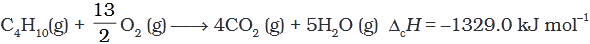(3)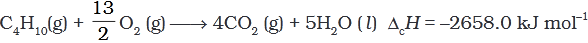(3)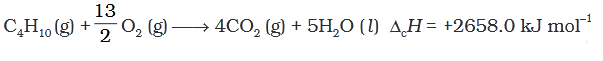Ans. (3)
Solution.
Standard enthalpy of combustion is defined as the enthalpy change per mole (or per unit amount) of a substance, when it undergoes combustion and all the reactants and products being in their standard states at the specified temperature.

Q.6. ∆fUΘ of formation of CH4 (g) at certain temperature is –393 kJ mol–1. The value of fHΘ is
(1) zero
(2) < ∆f UΘ
(3) f UΘ
(4) equal to f UΘ

Ans. (2)
Solution.
fH = fUΘ + ngRT
For the reaction,
C(s) + 2H2(g) → CH4(g)
ng = 1 - 2 = -1
fHΘ = fUΘ - 1 RT
or fHΘ = fUΘ + RT
or fHΘ < fUΘ

Q.7. In an adiabatic process, no transfer of heat takes place between system and surroundings. Choose the correct option for free expansion of an ideal gas under adiabatic condition from the following.
(1) q = 0, ∆T ≠0, w = 0
(2) q ≠ 0, ∆T =0, w = 0
(3) q = 0, ∆T =0, w = 0
(4) q = 0, ∆T <0, w ≠ 0
Ans. (3)
Solution.
For free expansion w = 0 for adiabatic process q = 0 from first law of thermodynamics,
U = q + w
= 0 + 0 = 0
Since there is no change of internal energy, hence temperature will also remain constant, i.e., ∆T = 0

Q.8. The pressure-volume work for an ideal gas can be calculated by using the expression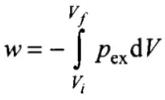The work can also be calculated from the pV– plot by using the area under the curve within the specified limits. When an ideal gas is compressed
(a) reversibly or (b) irreversibly from volume Vi to Vf. choose the correct option.
(1) w(reversible) = w (irreversible)
(2) w(reversible) < w (irreversible)
(3) w(reversible) > w (irreversible)
(4) w(reversible) = w (irreversible) + pex.∆V
Ans. (2)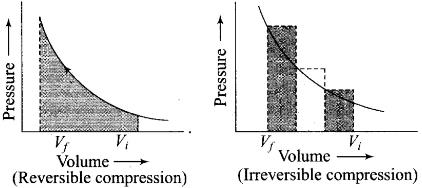Area under the curve is greater in irreversible compression than that of reversible compression.

Q.9. The entropy change can be calculated by using the expression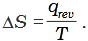When water freezes in a glass beaker, choose the correct statement amongst the following :
(1) ∆S (system) decreases but ∆S (surroundings) remains the same.
(2) ∆S (system) increases but ∆S (surroundings) decreases.
(3) ∆S (system) decreases but ∆S (surroundings) increases.
(4) ∆S (system) decreases and ∆S (surroundings) also decreases.
Ans. (3)
Solution.
During the process of freezing energy is released, which is absorbed by the surroundings.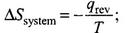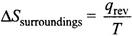Therefore, the entropy of the system decreases and that of surroundings increases.

Q.10. On the basis of thermochemical equations (a), (b) and (c), find out which of the algebraic relationships given in options (i) to (iv) is correct.
(a)
C (graphite) + O2(g) → CO2 (g) ;ΔrH = x kJ mol-1
(b)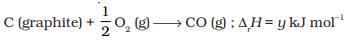(c)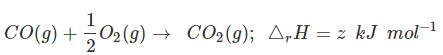(1) z = x + y
(2) x = y – z
(3) x = y + z
(4) y = 2z – x

Ans. (3)
Solution.
C (graphite) + O2(g) → CO2 (g) ;ΔfH = x kJ mol-1 ...(i)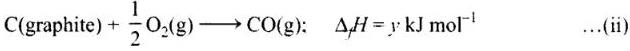Subtracting equation (i) and (ii) we get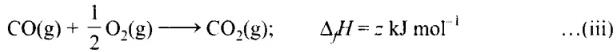Equation (iii) is obtained by subtracting (ii) from (i)
Therefore,
z = x - y
or x = y + z

Q.11. Consider the reactions given below. On the basis of these reactions find out which of the algebraic relations given in options (i) to (iv) is correct?
(a) C(g) + 4H(g) → CH4(g); ∆rH = x kJ mol–1
(b) C(graphite,s) + 2H2(g) → CH4(g); ∆rH = y kJ mol–1
(1) x = y
(2) x = 2y
(3) x > y
(4) x < y

Ans. (3)
Solution.
Same bonds are formed in reaction (a) and (b) but bonds between the reactant molecules are broken only in reaction (b).

Q.12. The enthalpies of elements in their standard states are taken as zero. The enthalpy of formation of a compound
(1) Is always negative
(2) Is always positive
(3) May be positive or negative
(4) Is never negative

Ans. (3)
Solution.
Heat of formation of a compound may be positive or negative,
Example,
C(s) + O2(g) → CO2(g);
ΔHº = -393.5 kJ mol-1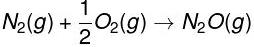ΔHº = +92 kJ mol-1

Q.13. Enthalpy of sublimation of a substance is equal to
(1) Enthalpy of fusion + enthalpy of vapourisation
(2) Enthalpy of fusion
(3) Enthalpy of vapourisation
(4) Twice the enthalpy of vapourisation
Ans.
(1)
Solution.

Enthalpy of sublimation of a substance is equal to enthalpy of fusion + enthalpy of vapourisation.
Sublimation is direct conversion of solid to vapour, i.e., solid → vapour
Writing in two steps, we have solid → liquid → vapour.
Solid → liquid requires enthalpy of fusion.
Liquid →vapour requires enthalpy of vapourisation.

Q.14. Which of the following is not correct?
(1) ∆G is zero for a reversible reaction
(2) ∆G is positive for a spontaneous reaction
(3) ∆G is negative for a spontaneous reaction
(4) ∆G is positive for a non-spontaneous reaction

Ans. (2)
Solution.
∆G gives a criterion for spontaneity at constant pressure and temperature.
(a) If ∆G is negative (< 0). the process is spontaneous.
(b) If ∆G is positiv e (> 0). the process is non-spontaneous.
(c) If ∆G is zero then reaction is at equilibrium.

Q.15. Thermodynamics mainly deals with
(1) Interrelation of various forms of energy and their transformation from
one form to another.
(2) Energy changes in the processes which depend only on initial and final
states of the microscopic systems containing a few molecules.
(3) How and at what rate these energy transformations are carried out.
(4) The system in equilibrium state or moving from one equilibrium state to
another equilibrium state.
Ans. (1, 4)
Solution.
Thermodynamics deals with interrelation of various forms of energy and their transformation into each other. It also deals with thermal or mechanical equilibrium. However, if does not tell anything about the rate of reaction.

Q.16. In an exothermic reaction, heat is evolved, and system loses heat to the surrounding. For such system
(1) qp will be negative

(2) ∆rH will be negative
(3) qp will be positive
(4) ∆rH will be positive
Ans. (1, 2)
Solution.
In an exothermic reaction, heat is evolved, and system loses heat to the surroundings. Therefore, qp will be negative and ∆rH will also be negative.

Q.17. The spontaneity means, having the potential to proceed without the assistance of external agency. The processes which occur spontaneously are
(1) Flow of heat from colder to warmer body.
(2) Gas in a container contracting into one corner.
(3) Gas expanding to fill the available volume.
(4) Burning carbon in oxygen to give carbon dioxide.

Ans. (1, 4)
Solution.
Gas expands or diffuses in available space spontaneously, e.g., leakage of cooking gas gives smell of ethyl mercaptan spontaneously. Moreover, burning of carbon to CO2 is also spontaneous.

Q.18. For an ideal gas, the work of reversible expansion under isothermal condition can be calculated by using the expression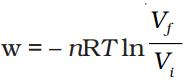A sample containing 1.0 mol of an ideal gas is expanded isothermally and reversibly to ten times of its original volume, in two separate experiments.
The expansion is carried out at 300 K and at 600 K respectively. Choose the correct option.
(1) Work done at 600 K is 20 times the work done at 300 K.
(2) Work done at 300 K is twice the work done at 600 K.
(3) Work done at 600 K is twice the work done at 300 K.
(4) ∆U = 0 in both cases.

Ans. (3, 4)
Solution..
Here,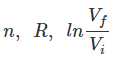are same in both the cases,
therefore,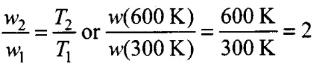i.e., work done at 600 K is twice the work done at 300 K. Since each case involves isothermal expansion of an ideal gas, there is no change in internal energy,
i.e., ∆U = 0.

Q.19. Consider the following reaction between zinc and oxygen and choose the correct options out of the options given below:
2Zn(s) + O2 (g) → 2ZnO (s) ; ∆H = – 693.8 kJ mol–1
(1) The enthalpy of two moles of ZnO is less than the total enthalpy of two
moles of Zn and one mole of oxygen by 693.8 kJ.
(2) The enthalpy of two moles of ZnO is more than the total enthalpy of two moles of Zn and one mole of oxygen by 693.8 kJ.
(3) 693.8 kJ mol–1 energy is evolved in the reaction.
(4) 693.8 kJ mol–1 energy is absorbed in the reaction.
Ans. (1, 3)
Solution.
In the reaction:
2Zn(s) + O2(g) → 2ZnO(s); ΔrH = -693.8 kJ mol-1
ΔrH =  ∑ Enthalpy of formation of products
-∑ Enthalpy of formation of reactants
= [2ΔfHZnO] - [2ΔfHZn + Δf HO2]
For exothermic reactions, ΔrH is negative, i.e., 693.8 kJ heat is evolved.
∴ Enthalpy of 2 moles of ZnO will less than the enthalpy of two moles of zinc and one mole of O2.

Q.20. 18.0 g of water completely vapourises at 100°C and 1 bar pressure and the enthalpy change in the process is 40.79 kJ mol–1. What will be the enthalpy change for vapourising two moles of water under the same conditions? What is the standard enthalphy of vapourisation for water?
Ans. Enthalpy of a reaction is the energy change per mole for the process.
18 g of H2O = 1 mole (∆Hvap = 40.79 kJ moE1)
Enthalpy change for vapourising 2 moles of H2O = 2 × 40.79 = 81.58 kJ ∆H°vap = 40.79 kJ mol -1

Q.21. One mole of acetone requires less heat to vapourise than 1 mol of water. Which of the two liquids has higher enthalpy of vapourisation?
Ans.
Due to weak force of attraction between molecules, acetone requires less heat to vaporise. Hence, water has higher enthalpy of vaporisation.

Q.22. Standard molar enthalpy of formation, ∆fHΘ is just a special case of enthalpy of reaction, ∆rHΘ. Is the ∆rHΘ for the following reaction same as ∆fHΘ? Give reason for your answer.
CaO(s) + CO2(g) → CaCO3(s); ∆fHΘ = –178.3 kJ mol
–1
Ans. No, standard molar enthalpy of formation is the enthalpy of the reaction when 1 mole of compound is formed from its constituent elements.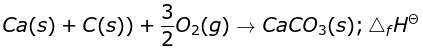As the close reaction is different from the given reaction,
∴ ΔrHΘ ≠ ΔfHΘ

Q.23. The value of ∆fHΘ for NH3 is –91.8 kJ mol–1. Calculate enthalpy change for the following reaction:
2NH3(g) → N2(g) + 3H2(g)

Ans.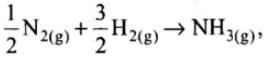ΔfHΘ= -91.8 kJ mol-1
or N2(g) + 3H2(g) → 2NH3(g),
ΔfHΘ  = -2 x 91.8 = -183.6 kJ mol-1
So, for the reverse reaction,
ΔrHΘ = 183.6 kJ mol-1.
∴ The value of ΔrHΘ for NH3 is +183.6 kJ mol-1

Q.24. Enthalpy is an extensive property. In general, if enthalpy of an overall reaction A→B along one route is ∆H and ∆rH1, ∆rH2, ∆rH3 ..... represent enthalpies of intermediate reactions leading to product B. What will be the relation between ∆rH for overall reaction and ∆rH1 , ∆rH2 ..... etc. for intermediate reactions.
Ans. By Hess’s law ∆rH = ∆rH+ ∆rH2 + ∆rH3……………………

Q.25. The enthalpy of atomisation for the reaction CH4(g) → C(g) + 4H (g) is 1665 kJ mol–1. What is the bond energy of C–H bond?
Ans.
H4 → C + 4H, ∆H= + 1665 kJ mol-1
Bond energy of (C – H) bond = 1665/4 = 416.2 kJ mol

Q.26. Use the following data to calculate ∆latticeHΘ for NaBr.
subHΘ for sodium metal = 108.4 kJ mol–1
Ionization enthalpy of sodium = 496 kJ mol–1
Electron gain enthalpy of bromine = – 325 kJ mol–1
Bond dissociation enthalpy of bromine = 192 kJ mol–1
fHΘ for NaBr (s) = – 360.1 kJ mol–1
Ans.
(i) Na(s) → Na(g), ΔsubHΘ = 108.4 kJ mol-1
(ii) Na Na+ + e-, ΔiHΘ = 496 kJ mol-1
(iii) 1/2 Br2 → Br, 1/2 ΔdissHΘ = 192/2 = 96 kJ mol-1
(iv) Br + e- → Br-, ΔegHΘ = -325 kJ mol-1
ΔfHΘ for NaBr = -360.1 kJ mol -1
Enthalpies in different steps involved in the formation of NaBr(s) are.
ΔfHΘ = ΔsubHΘ + 1/2 ΔsubHΘ + ΔiHΘ + ΔegHΘ + ∆latticeHΘ
∴ ∆latticeHΘ = - 360.1 - 108.4 - 96 - 496 + 325
= -735.5 kJ mol-1

Q.27. Given that ∆H = 0 for mixing of two gases. Explain whether the diffusion of these gases into each other in a closed container is a spontaneous process or not?
Ans. It is a spontaneous process. Although enthalpy change is zero but randomness or disorder (ΔS) increases and ΔS is positive. Therefore, in equation, ΔG = ΔH – TΔS, the term TΔS will be negative. Hence ΔG will be negative.

Q.28. Heat has randomising influence on a system and temperature is the measure of average chaotic motion of particles in the system. Write the mathematical relation which relates these three parameters.
Ans. Heat has randomising influence on a system and temperature is the measure of average chaotic motion of particles in the system. The mathematical relation which relates these three parameters is ΔS = qrev/ T
Here, ΔS = change in entropy
qrcv = heat of reversible reaction
T = temperature

Q.29. Increase in enthalpy of the surroundings is equal to decrease in enthalpy of the system. Will the temperature of system and surroundings be the same when they are in thermal equilibrium?
Ans. Yes. If the system is in thermal equilibrium with the surroundings, then the temperature of the surroundings is same as that of the system. Also, increase in enthalpy of the surroundings is equal to decrease in the enthalpy of the system.

Q.30. At 298 K. Kp for the reaction N2O4 (g) ⇌ 2NO2 (g) is 0.98. Predict whether the reaction is spontaneous or not.
Ans.
ΔrGΘ = -RT ln Kp
= -RT ln (0.98)
Since In (0.98) is negative
∴ ΔrGΘ is positive
⇒ the reaction is non spontaneous

Q.31. A sample of 1.0 mol of a monoatomic ideal gas is taken through a cyclic process of expansion and compression as shown in Figure. What will be the value of ∆H for the cycle as a whole?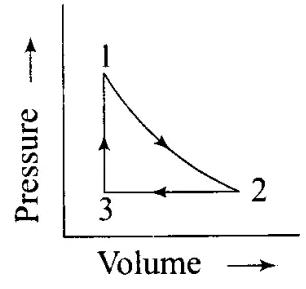Ans. For a cyclic process, ΔH = 0

Q.32. The standard molar entropy of H2O (l) is 70 J K–1 mol–1. Will the standard molar entropy of H2O(s) be more, or less than 70 J K–1 mol–1?
Ans. The standard molar entropy of H2O (1) is 70 J K-1 mol-1. The solid form of H2O is ice. In ice, molecules of H2O are less random than in liquid water. Thus, molar entropy of H2O (s) < molar entropy of H2O (1). The standard molar entropy of H2O (s) is less than 70 J K1 mol-1.

Q.33. Identify the state functions and path functions out of the following: enthalpy, entropy, heat, temperature, work, free energy.
Ans. State functions: Enthalpy, entropy, temperature, free energy Path functions: Heat, work.

Offer running on EduRev: Apply code STAYHOME200 to get INR 200 off on our premium plan EduRev Infinity!

233 docs

,

,

,

,

,

,

,

,

,

,

,

,

,

,

,

,

,

,

,

,

,

;